7. ALTERNATING CURRENT

Ans ⇒ Advantages of a.c. over d.c. :
(i) It is economical to generate a.c. than d.c.

(ii) A.C.voltage can be easily stepped up or stepped down with the help of a transformer.

(iii) It is easy to transfer high a.c. voltage to distant places at a small loss of electric power.

(iv) A.C. can be easily converted into d.c. by using a rectifier.

(v) The alternating currents can be easily regulated by using a choke coil without loss of electric energy.

Disadvantages of a.c. over d.c. :
(i) It cannot be used in electrolytic processes such as electrolyping, electroplating etc.

(ii) A. C. supply is more dangerous and fatal than d.c. due to the shock produced by it.

(iii) To transmit a.c., a number of thin wires insteads of a thick single wire are used so as to avoid skin effect.

Q.2. Distinguish between resistance, impedance and reactance of an a.c. circuit.

Ans ⇒ Difference between resistance, impedance and reactance of an a.c. circuit are as follow :

 S.N Resistance Impedance Reactance 1. It is defined as the opposition offered by the resistor to the flow of current. It is defined as the opposition offered by the circuit containing resistor,inductor and the capacitor to the flow of current. It is defined as the opposition offered by the capacitor or inductor to the flow of current. 2. It is independent of the frequency of the source of current. It depends upon the frequency of the source of current. It depends on the frequency of the source of the current. 3. It is measured in ohm. It is measured in ohm. It is measured in ohm.

Q.4. Explain the use of a choke coil or alternator of a motor.

Ans ⇒ Choke coil : It is an induction coil of low resistance which reduces the high value of alternating current (a.c.) without any loss of heat energy.
It is used in a tube light in the series so that the tube light is protected from the initial high value of current.

Alternator : It is a safety device connected in series of a motor. The initial current of a motor is 6 or 8 times the average value. The motor alternater reduces this value to normal level. Thus motor is safe.

Q.5. Why is a choke coil needed in the use of fluorescent tubes with a.c. mains ? Why can we not use an ordinary resistor instead of the choke coil ?

Ans ⇒ For choke coil, φ = π/2
Average power consumed
Pav = Iv Evcosφ = IvEcosπ/2
Pav = O and XL = ωL = 2πvL

Hence ωL can control a.c. with consuming power.
For ordinary resister φ = 0
∴    P = Iv Ev cosφ = Iv Ev
and impedence = R
Therefore, resistor consume maximum power. Hence a choke is used in fluorescent tubes with a.c. main than an ordinary resistor.

Q.6. Show that the angular frequency of free oscillations of an LC circuit is equation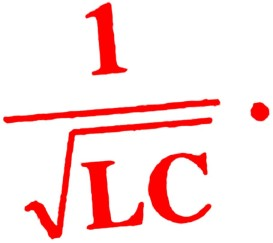Ans ⇒ Suppose a capacitor C has a charge q0 at t = 0 and is connected to an inductance as shown in Fig. The charge on capacitor decreases and there is current in circuit.
Let at time t, q and I be charge and current in circuit.

Here dl/dt is positive.
Voltage across C at time t = q/c and induced voltage across inductance voltage will be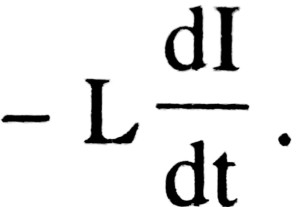By Kirchhoff’s second law since supply voltage is zero. Therefore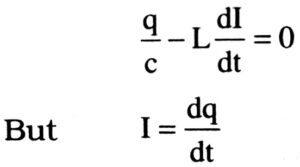Negative sign shows that as q decreases I increases.
Putting value of I from (ii) in (i)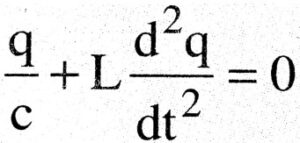Dividing both sides by L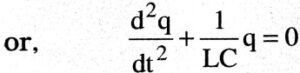This is of differential equation type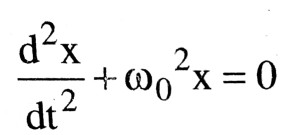Comparing (ii) and (iv)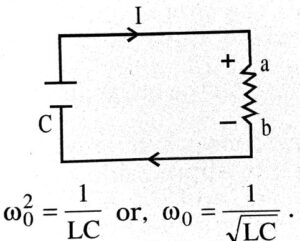Q.7. Show that in the free oscillations of an LC circuit, the sum of energies stored in the capacitor and the inductor is constant in time.

Ans ⇒ Energy stored in a capacitor at any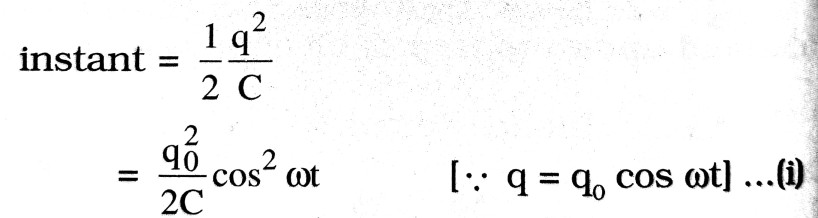Energy stored in an inductor at any instant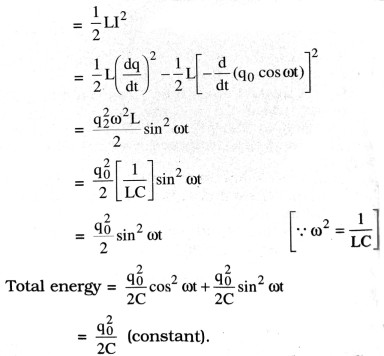Q.8. An a.c. source ε = ε0 sin ot is applied across an inductance L obtain an expression for the current.

Ans ⇒ Alternating e.m.f. applied across inductor is represented as
ε = ε0 sin ωt
Let the inductor be a pure inductor of inductance L. If dl/dt is the rate of change of current through L at any instant, then induced e.m.f. in the inductor is equal to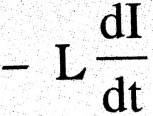(the negative sign indicates that induced e.m.f. opposes the change of current). To maintain the flow of current, applied e.m.f. must be equal and opposite to the induced e.m.f.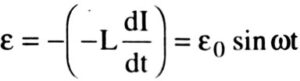Or dl = sin ωt dt
Integratiting both sides, we get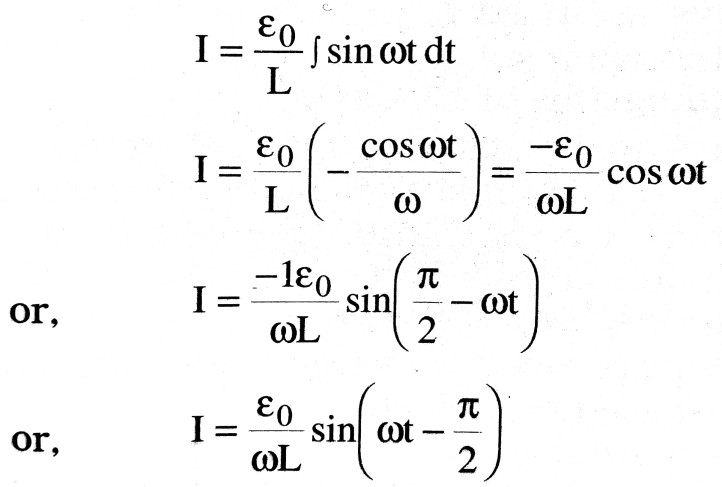The current is maximum when sin(ωt – π/2) = 1 = maximum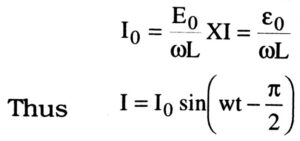Comparing Eq. (iii) with Ohm’s law, I = V/R and denoting the effective resistance or inductive reactance by XL,we get

XL = ωL
Eq. (iv) is the required relation.

Q.9. What is a choke coil ? Why is it preferred to resistance in a.c. circuit ?
In figures (a), (b) and (c) three a.c. circuits with equal currents have been shown.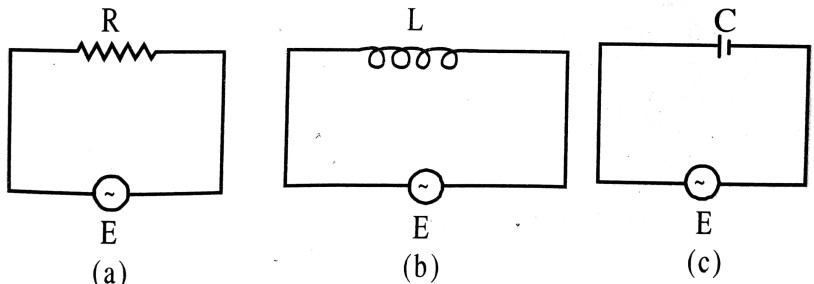If the frequency of e.m.f. be increased, then what will be the effect on the currents flowing in them ? Explain with reason.

Ans ⇒ Choke coil : It is a high inductance coil made of thick insulated copper wire wound closely in a large number of turns over a soft iron laminated core.
Choke coils reduces current without wasting electrical energy in the form of heat.
(i) There is no effect in ‘a’ as the current flowing in the coil as R is not affected by the frequency change.
(ii) Current will decrease in (b) because inductive reactance XL = 2πvL and it increases with increase in frequency.
(iii) Current will decrease in (c) because capacitive reactance
Xc = (2πvC)-1
As Xc ∝ 1/v
It will decrease with increase in frequency.

Q.10. Derive an expression for the impedance of an a.c. circuit with an inductor and a resistor in series.

Ans ⇒ Let an alternating emf ε0 = ε sin ωt
is applied across a series combination of an inductor and a resistor as shown in Fig. The inductance of the inductor is L and the resistance of the resistor is R.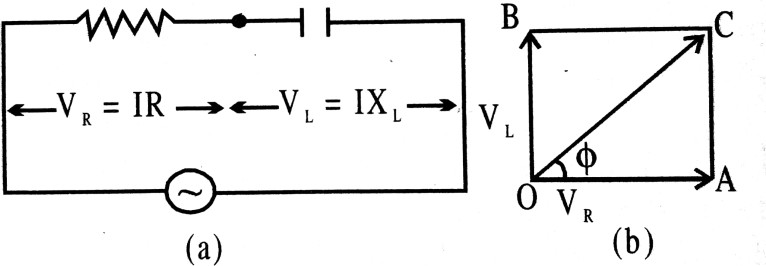Since L and Rare in series, same current I flows through them. However, the voltage across them are different.
Voltage across the resistor
VR = IR
Voltage across the inductor
VL = IXL
As shown in Fig. VR is in phase with current I and is represented by vector OA along positive x-axis While VL is ahead of the current I by a phase angle of

π/2 radian and is represented by vector OB along positive y = axis.

The resultant voltage V is represented by vector OC and is found by parallelogram law of vector addition.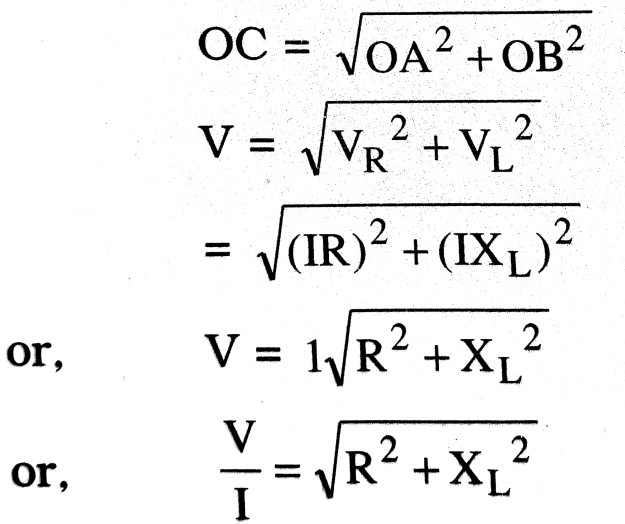Comparing the above expression with Ohm’s law, we find that the effective resistance or impedance (2) offered by a series LR-circuit is given by :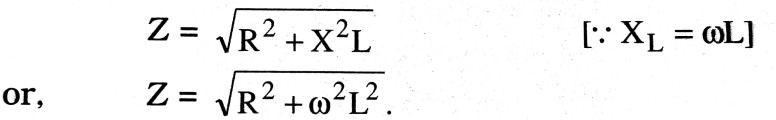Q.11. Show that the average value of a.c. over a complete cycle is zero while for a half. cycle it is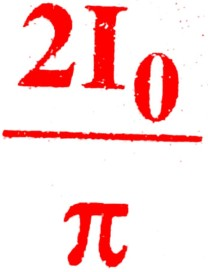where I0 is the peak value of current.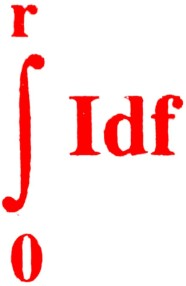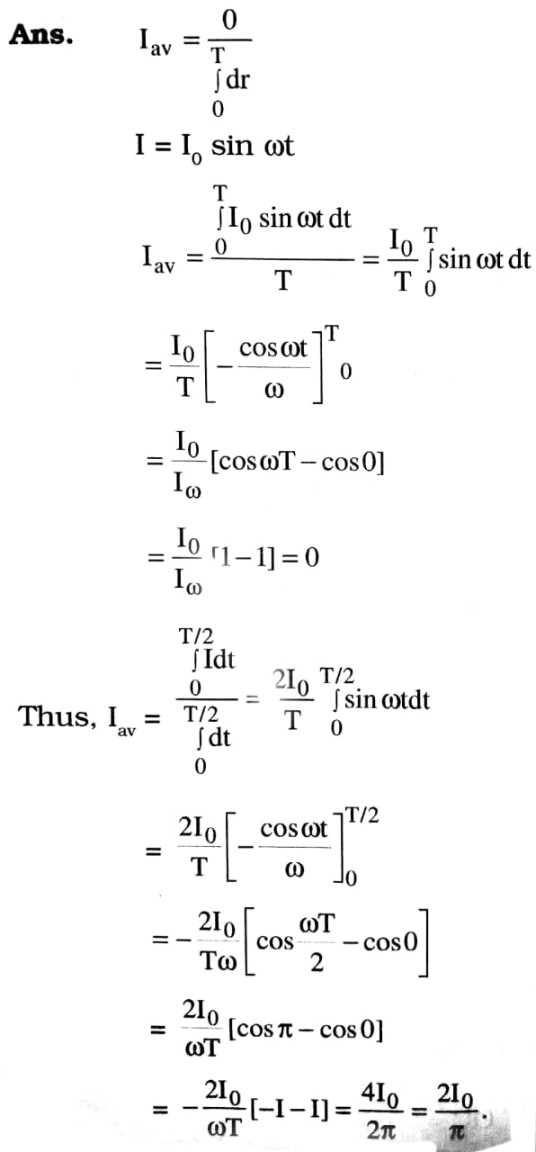## Class 12th physics Subjective question in English

 S.N Physics Short Type Question English Medium 1. ELECTRIC CHARGES AND FIELDS 2. LECTROSTATIC POTENTIAL AND CAPACITANCE 3. CURRENT ELECTRICITY 4. MOVING CHARGES AND MAGNETISM 5. MAGNETISM AND MATTER 6. ELECTROMAGNETIC INDUCTION 7. ALTERNATING CURRENT 8. ELECTROMAGNETIC WAVES 9. RAY OPTICS AND OPTICAL INSTRUMENTS 10. WAVE OPTICS 11. DUAL NATURE OF MATTER AND RADIATION 12. ATOMS 13. NUCLEI 14. SEMI CONDUCTOR ELECTRONICS 15. COMMUNICATION SYSTEMS